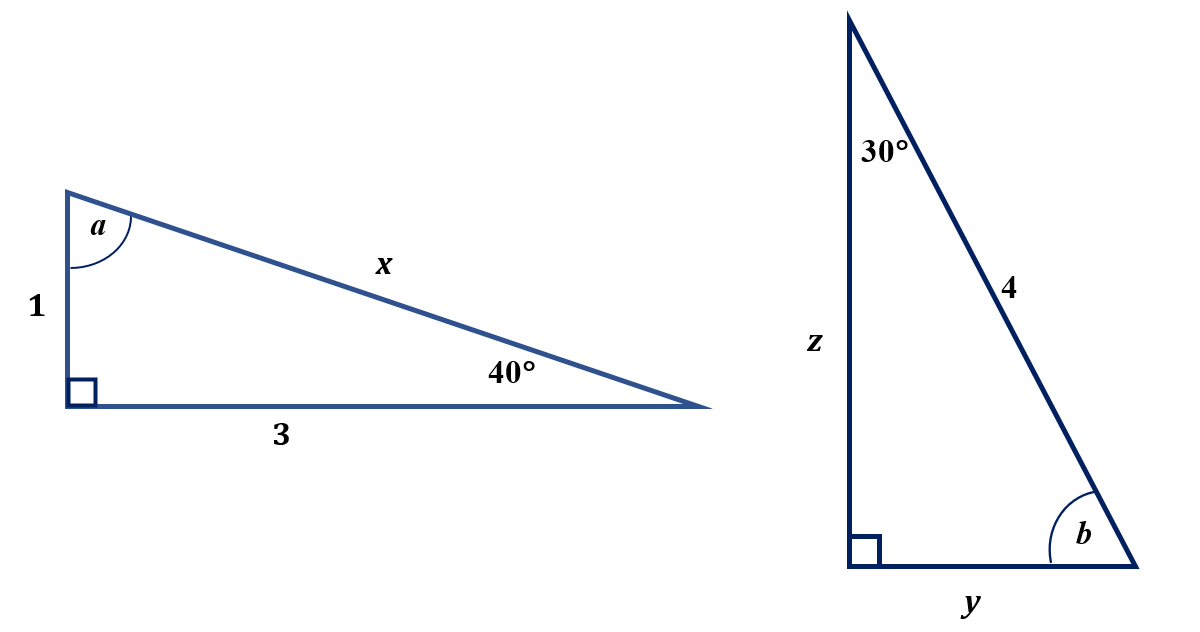# GEOM 2 | Lesson 4 | Practice (Finding Triangle Parts)In Making Connections, the sine, cosine, and tangent are the same for triangles with different side length and with angles of 30, 60, and 45.  This is true based on the following definitions.

DEFINITION: Triangles with the same angles but different lengths are similar (same shape but different size) and produce the same ratio when certain sides are divided.

DEFINITION:  Ratios of certain sides in two different triangles with the same angles are congruent (similarity ratios).

## Practice:  Finding Missing Parts

• Find the missing parts of each triangle (angles and sides) using the Pythagorean Theorem, the Triangle Sum Principle, and special right triangle relationships.
• Then write the trig ratios.  Leave your answers in fraction form (note, be sure to rationalize the denominator when applicable).1. $$\angle a=$$
2. $$x=$$
3. sin $$40^\circ$$=
4. tan $$a=$$
5. cos $$40^\circ$$=
6. $$\angle b=$$
7. $$y=$$
8. $$z=$$
9. sin $$30^\circ$$=
10. tan $$b=$$
11. cos $$30^\circ$$=

Check solutions here.